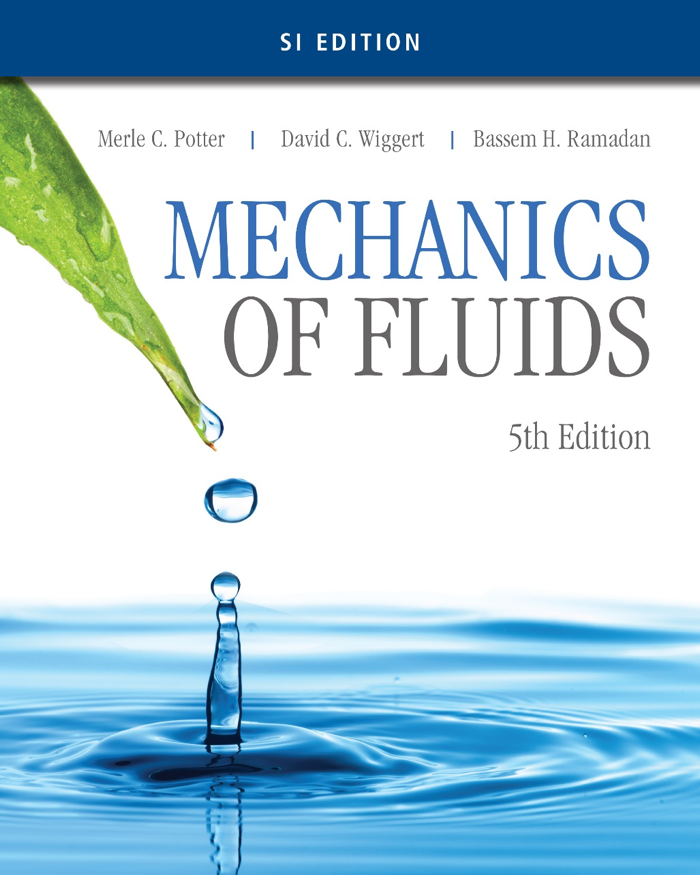• ### COURSE INFORMATION

Open or Close
The principle aim of this course is to provide students with an understanding of the properties of fluids and to introduce fundamental laws and description of fluid behavior and flow. It will emphasize on the concept of pressure, hydrostatic pressure equation and its application in the measurement of pressure, static force due to immersed surfaces, floatation and buoyancy analysis. Dynamic flow analysis inclusive of technique in solving flow problems is introduce especially to solve flow measurement, mass or volumetric flow rate, momentum in flow and loss in pipe network. Lastly, some basic dimensional analysis and similarities will be introduced. At the end of the course, the student should be able to demonstrate an ability to analyze whether statically, dynamically or kinematically problems related directly to fluids.

COURSE LEARNING OUTCOMES
1. Calculate and analyse shear stress in fluids flow, the pressure difference in manometer, hydrostatic forces on plane and curved surfaces, buoyancy force and stability of submerged and floating bodies.
2. Calculate and analyse fluid dynamics problems using integral forms and differential forms of three basic laws: Bernoulli, energy and momentum equation.
3. Categorize, calculate and analyse the major and minor losses for laminar and turbulent flows in pipes and pipes systems.
4. Derive the dimensionless parameters for a given flow problem using dimensional analysis and similitude in order to solve various problems related to modelling.
• ### SYLLABUS

Open or Close
Overview of course
Introduction:
Definition of fluid; Dimension & unit fluid properties.

Fluid Statics:
Pascal’s law, pressure variations fluid at rest – pressure measurements.
Forces on plane and curve surface.
Buoyancy. Linearly accelerating containers.

Introduction to fluids in motion:
Description of fluid Motion, classification of fluid flows.
The Bernoulli equation.
Fundamental laws – Integral form.
Control volume, conservation of mass.
Momentum equation – Deflectors, propellers.
Fundamental laws – Differential forms.
Continuity equation
Momentum equation, energy equation.

Dimensional analysis:
Buckingham Pi-theorem.
Similitude – confined flows, free-surface flows, high Reynold-number flows.
Normalized differential equations.

Internal flow:
Laminar flow in a pipe
Turbulent flow in a pipe – Major and minor losses, hydraulic and energy grade lines. Simple pipe system with a pump.

NOTES

• ### ASSESSMENT

Open or Close
Test 1 - 20%
Test 2 - 20%

Assignment - 20%

Final Exam - 40%

(Marks percentage may be changed due to Fluid Panel's decision)
• ### REFERENCES

Open or CloseOfficial Text book
Potter, Wiggert, Ramadan. 2016. Mechanics of Fluids, SI Edition, 5th Ed. Cengage Learning.
Other References
1. Munson, Young, Okiishi, Huebsch. 2010. Fundamental of Fluid Mechanics, 6th Ed. John Wiley and Sons.
2. Fox, McDonald and Pritchard, 2004, Introduction to Fluid Mechanics, 6th Edition, John Wiley and Sons.
3. Douglas, Gasiorek, Swaffield, Fluid Mechanics, 4th Edition, Prentice Hall.
4. White. 2016. Fluid Mechanics. 8th Edition. McGraw-Hill International Edition.
5. Massey, Mechanics of Fluids, Chapman & Hall, London.